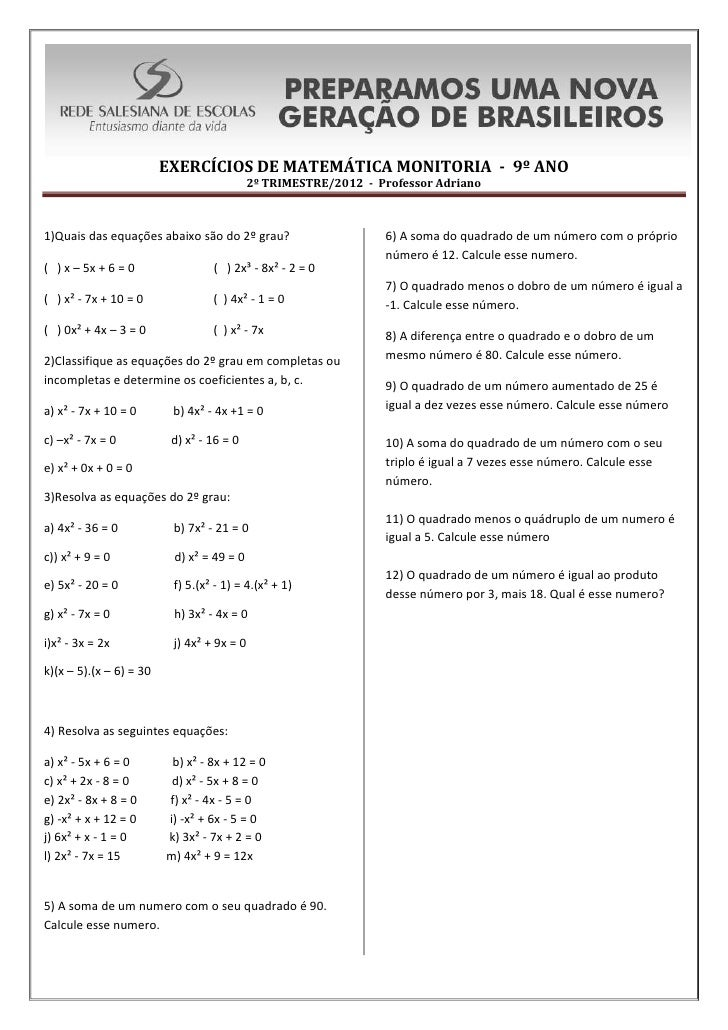Successfully reported this slideshow.Upcoming SlideShare
×

# Exercícios de matemática monitoria 9ano cópia

• Full Name
Comment goes here.

Are you sure you want to Yes No• Be the first to comment

### Exercícios de matemática monitoria 9ano cópia

1. 1. EXERCÍCIOS DE MATEMÁTICA MONITORIA - 9º ANO 2º TRIMESTRE/2012 - Professor Adriano1)Quais das equações abaixo são do 2º grau? 6) A soma do quadrado de um número com o próprio número é 12. Calcule esse numero.( ) x – 5x + 6 = 0 ( ) 2x³ - 8x² - 2 = 0 7) O quadrado menos o dobro de um número é igual a( ) x² - 7x + 10 = 0 ( ) 4x² - 1 = 0 -1. Calcule esse número.( ) 0x² + 4x – 3 = 0 ( ) x² - 7x 8) A diferença entre o quadrado e o dobro de um2)Classifique as equações do 2º grau em completas ou mesmo número é 80. Calcule esse número.incompletas e determine os coeficientes a, b, c. 9) O quadrado de um número aumentado de 25 éa) x² - 7x + 10 = 0 b) 4x² - 4x +1 = 0 igual a dez vezes esse número. Calcule esse númeroc) –x² - 7x = 0 d) x² - 16 = 0 10) A soma do quadrado de um número com o seue) x² + 0x + 0 = 0 triplo é igual a 7 vezes esse número. Calcule esse número.3)Resolva as equações do 2º grau: 11) O quadrado menos o quádruplo de um numero éa) 4x² - 36 = 0 b) 7x² - 21 = 0 igual a 5. Calcule esse númeroc)) x² + 9 = 0 d) x² = 49 = 0 12) O quadrado de um número é igual ao produtoe) 5x² - 20 = 0 f) 5.(x² - 1) = 4.(x² + 1) desse número por 3, mais 18. Qual é esse numero?g) x² - 7x = 0 h) 3x² - 4x = 0i)x² - 3x = 2x j) 4x² + 9x = 0k)(x – 5).(x – 6) = 304) Resolva as seguintes equações:a) x² - 5x + 6 = 0 b) x² - 8x + 12 = 0c) x² + 2x - 8 = 0 d) x² - 5x + 8 = 0e) 2x² - 8x + 8 = 0 f) x² - 4x - 5 = 0g) -x² + x + 12 = 0 i) -x² + 6x - 5 = 0j) 6x² + x - 1 = 0 k) 3x² - 7x + 2 = 0l) 2x² - 7x = 15 m) 4x² + 9 = 12x5) A soma de um numero com o seu quadrado é 90.Calcule esse numero.

### Be the first to comment

•#### pa689064

Sep. 10, 2012
•#### jocilenesilva6102

Oct. 23, 2016

Total views

14,494

On Slideshare

0

From embeds

0

Number of embeds

3

165

Shares

0